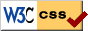# Notes on Diffy Qs - SAGE demonstrations for section 1.6

Press the Activate buttons below to launch the SAGE demonstration. After the sage cell is evaluated you should be able to interact with it to change numbers with sliders. You may have to wait a little before the graph appears. Be patient.

## Logistic equation

$x' = -k x(x-A)$.

A plot of the slope field is shown, the two equilibria solutions are plotted in green. Three representative solutions are plotted in blue. Finally, a solution with the initial value $x(0) = I$ is plotted in red. Sliders are presented for the values of $k$, $A$, and $I$.

## Exponential growth/decay

Let us now look at the exponential growth and decay:

$x' = k(x-A)$, $x(0)= I$.

This cell will plot the above exponential growth and decay. The values of $k$, $A$, and $I$ are changable with the sliders. In this plot, we will also draw the phase diagram to the right of the plot.

$x' = -kx(x-A) + B$, $x(0)= I$.